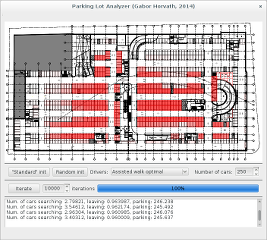# Software tools corresponding to the papers

## "Transient and First Passage Time Distributions of First- and Second-order Multi-regime Markov Fluid Queues via ME-fication"

The MATLAB implementation and the numerical examples presented in the paper can be downloaded from this link.

## "Matrix-analytic solution of infinite, finite and level-dependent second-order fluid models"

The MATLAB implementation and the numerical examples presented in the paper can be downloaded from this link.

## "Waiting time and queue length analysis of Markov-modulated fluid priority queues"

The MATLAB implementation and the numerical examples presented in the paper can be downloaded from this link.

## "Queue length analysis of a Markov-modulated vacation queue with dependent arrival and service processes and exhaustive service policy"

The Mathematica implementation and the code used for the numerical examples can be downloaded from this link. BuTools V2.0 is required to be installed.

## "Analysis of QBD queues with matrix-geometrically distributed batch arrivals and services"

The MATLAB implementation of the procedures presented in the paper and the code corresponding to the numerical examples can be downloaded from this link.

Required dependency:

• SMCSolver for QBDs (from here). Add the SMCSolver/QBD directory to the MATLAB path.

## "Measuring the distance between MAPs and some applications"

The MATLAB implementation of the procedures presented in the paper and the code corresponding to the numerical examples can be downloaded from this link.

Two dependencies are required:

• PHCpack and PHClab (from here). Add the PHClab directory to MATLAB, and run the set_phcpath function with the path to the phc binary.
• SMCSolver for QBDs (from here). Add the SMCSolver/QBD directory to the MATLAB path.

## "Efficient Analysis of the MMAP[K]/PH[K]/1 Priority Queue"

The MATLAB implementation of the procedures presented in the paper and the code corresponding to the numerical example can be downloaded from this link.

## "Moment Matching-Based Distribution Fitting with Generalized Hyper-Erlang Distributions"

The MATLAB implementation of the moment matching method can be downloaded from this link (example is included). This procedure requires the PHCpack tool and PHClab, both are available for free at the homepage of the author.

## "Sojourn Times in Fluid Queues with Independent and Dependent Input and Output Processes"

The MATLAB implementation of the procedures presented in the paper and the code corresponding to the numerical example can be downloaded from this link.

These procedures will be the parts of the soon to be released BuTools V2

## "A fast EM algorithm for fitting marked Markovian arrival processes with a new special structure"

The fitting tool implementing the algorithms of this paper is called spemfit. The corresponding source code can be downloaded from this link.

Note that this tool also implements the G-FIT procedure as well, and since it makes use of multi-core cpus it is faster than the one developed by the authors.

This procedure will be the part of the soon to be released BuTools V2

## "Matching marginal moments and lag autocorrelations with MAPs"

Implementation including the example test cases:

This procedure will be the part of the soon to be released BuTools V2

## "Performance Modeling of Intelligent Car Parking Systems"

The source code corresponding to this paper is located in this bitbucket repository (it uses the cross-platform Qt toolkit).Usage: Enter the number of cars Select driver behavior model Press either the "Standard" init, or the "Random" init button Enter the number of iterations to take Start iterations with the "Iterate" button Status info appears in the window, the performance measures are printed to the standard output If more iterations are required, you can press "Iterate" again without re-initializing

# Other software projects

RawTherapee is an open source image processing software for digital photography. The development was started by me in 2005. I was the sole developer till version 3.0. In 2010 I decided to open the source code and gradually stopped contributing the project. Fortunately the development did not stop, many talented volunteers got involved and rawtherapee keeps improving faster than ever.